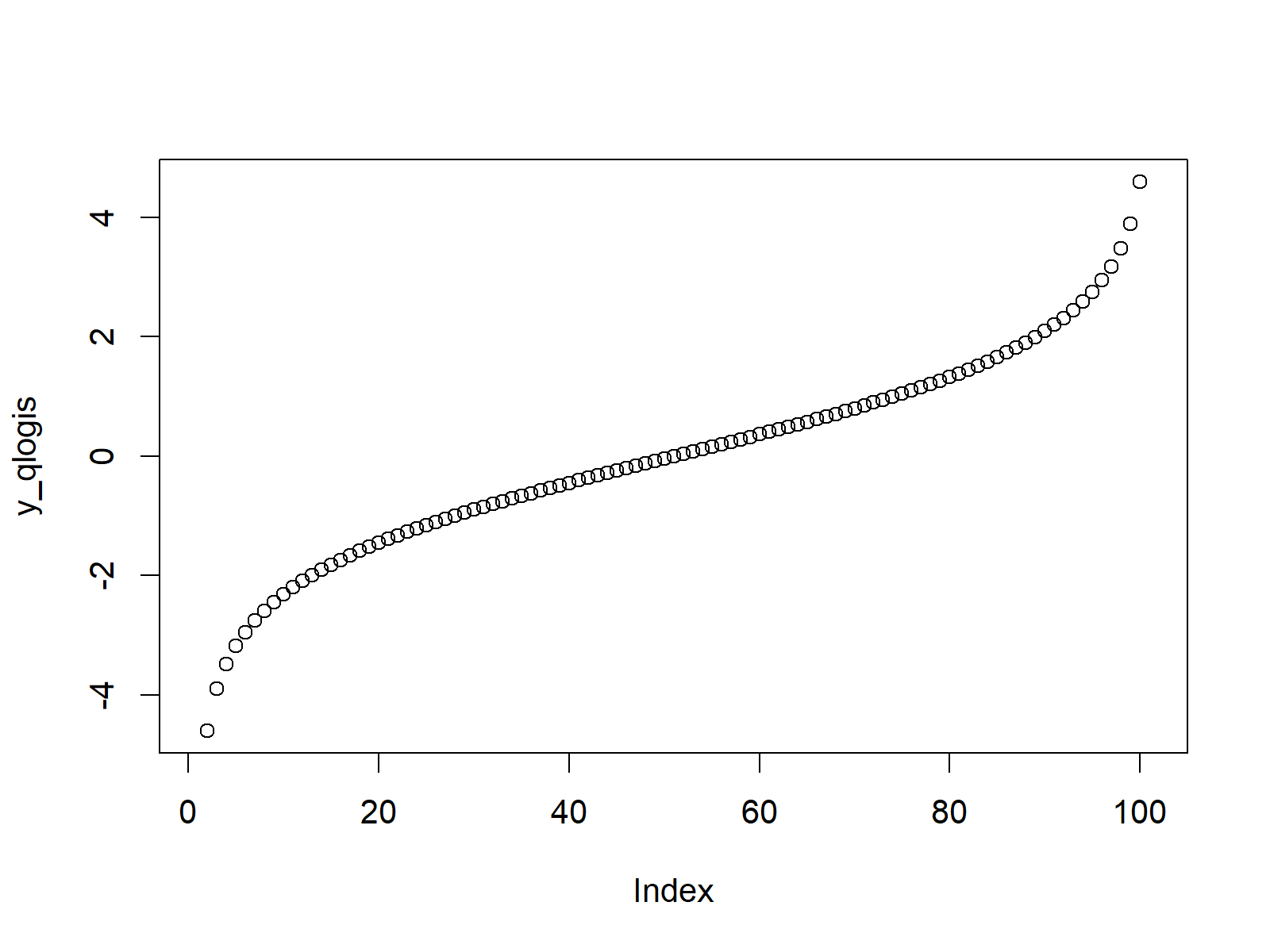# Logistic Distribution in R (4 Examples) | dlogis, plogis, qlogis & rlogis Functions

In this R tutorial you’ll learn how to apply the logistic functions.

The article contains this information:

Let’s take a look at some R codes in action…

## Example 1: Logistic Density in R (dlogis Function)

Let’s start with the density of the logistic distribution in R. First, we have to create a sequence of quantiles:

`x_dlogis <- seq(- 10, 10, by = 0.1)                     # Specify x-values for dlogis function`

Then, we can insert these quantiles into the dlogis function as you can see below:

`y_dlogis <- dlogis(x_dlogis)                            # Apply dlogis function`

To visualize the output of the dlogis function, we can draw a plot of its output:

`plot(y_dlogis)                                          # Plot dlogis values`Figure 1: Logistic Probability Density Function (PDF).

Figure 1 shows the logistic probability density function (PDF).

## Example 2: Logistic Cumulative Distribution Function (plogis Function)

In Example 2, we’ll create a plot of the logistic cumulative distribution function (CDF) in R. Again, we need to create a sequence of quantiles…

`x_plogis <- seq(- 10, 10, by = 0.1)                     # Specify x-values for plogis function`

…that we can use as input for the plogis function:

`y_plogis <- plogis(x_plogis)                            # Apply plogis function`

With the plot function, we can illustrate the output of plogis:

`plot(y_plogis)                                          # Plot plogis values`Figure 2: Logistic Cumulative Distribution Function (CDF).

## Example 3: Logistic Quantile Function (qlogis Function)

The R programming language also provides a command for the logistic quantile function. This time we need to create a sequence of probabilities as input:

`x_qlogis <- seq(0, 1, by = 0.01)                        # Specify x-values for qlogis function`

Now, we can use the qlogis R command to create the logistic quantile function:

`y_qlogis <- qlogis(x_qlogis)                            # Apply qlogis function`

As in the previous examples, we can illustrate the output with the plot function:

`plot(y_qlogis)                                          # Plot qlogis values`Figure 3: Logistic Quantile Function.

## Example 4: Generating Random Numbers (rlogis Function)

We can also generate a set of random numbers with a logistic distribution. First, we need to set a seed for reproducibility and a sample size of random numbers that we want to simulate:

```set.seed(91929)                                         # Set seed for reproducibility
N <- 10000                                              # Specify sample size```

Now, we can apply the rlogis function to draw a set of random numbers:

```y_rlogis <- rlogis(N)                                   # Draw N logistically distributed values
y_rlogis                                                # Print values to RStudio console
# -0.202638116  4.253454975 -0.546483563  1.015626074  0.713380391  1.228554727 -0.469496369  0.574367616...```

The following histogram shows the output of rlogis:

```hist(y_rlogis,                                          # Plot of randomly drawn logis density
breaks = 70,
main = "")```Figure 4: Logistic Random Numbers.

## Video, Further Resources & Summary

Would you like to know more about the logistic distribution in R? Then you may want to have a look at the following video of my YouTube channel. In the video instruction, I explain the content of this tutorial in RStudio.

You could also have a look at the other tutorials on distributions and the simulation of random numbers in R:

Besides the video, you could have a look at the related tutorials on this website. A selection of tutorials is listed here:

To summarize: At this point you should know how to draw and simulate a logistic distribution in the R programming language. In case you have further comments and/or questions, tell me about it in the comments section.

Subscribe to the Statistics Globe Newsletter